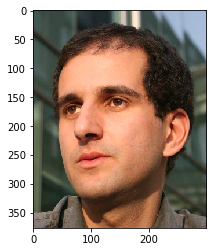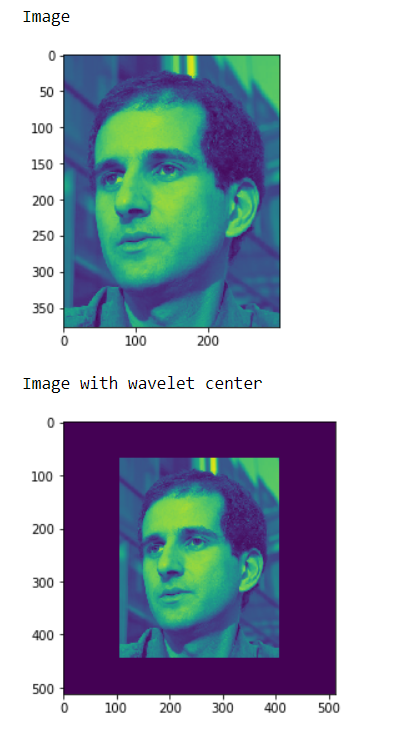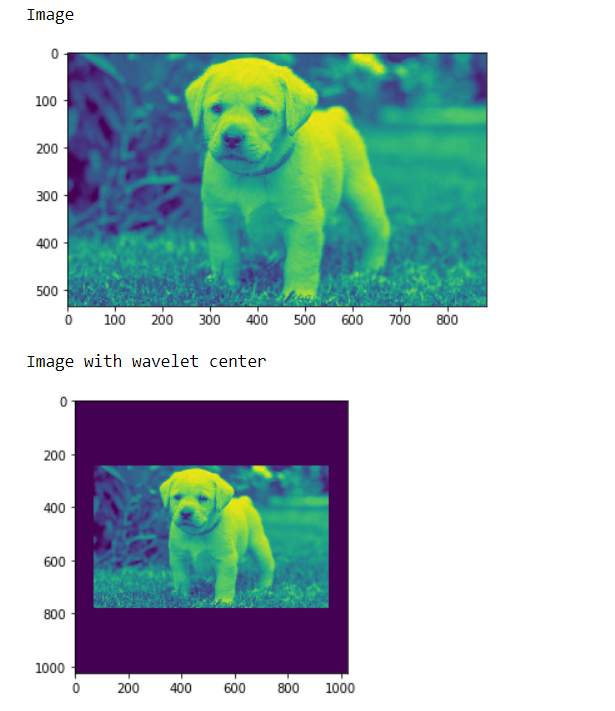# Mahotas – Making Image Wavelet Center

• Last Updated : 07 Jun, 2021

In this article we will see how we can make the image wavelet center in mahotas. Wavelets represent the scale of features in an image, as well as their position. Wavelet center will make the image small and place it in the center unlike daubechies wavelet.
In this tutorial we will use “luispedro” image, below is the command to load it.

`mahotas.demos.load('luispedro')`

Below is the luispedro imageIn order to do this we will use wavelet_center method

Syntax : mahotas.wavelet_center(img)
Argument : It takes image object as argument
Return : It returns image object

Note : Input image should be filtered or should be loaded as grey
In order to filter the image we will take the image object which is numpy.ndarray and filter it with the help of indexing, below is the command to do this

`image = image[:, :, 0]`

Example 1:

## Python3

 `# importing various libraries``import` `numpy as np``import` `mahotas``import` `mahotas.demos``from` `mahotas.thresholding ``import` `soft_threshold``from` `pylab ``import` `imshow, show``from` `os ``import` `path` `# loading image``f ``=` `mahotas.demos.load(``'luispedro'``, as_grey ``=` `True``)`  `# showing image``print``(``"Image"``)` `imshow(f)``show()` `# making image wavelet center``fc ``=` `mahotas.wavelet_center(f)` `# showing image``print``(``"Image with wavelet center"``)``imshow(fc)``show()`

Output :Example 2:

## Python3

 `# importing required libraries``import` `mahotas``import` `numpy as np``from` `pylab ``import` `imshow, show``import` `os`  `# loading image``img ``=` `mahotas.imread(``'dog_image.png'``)` `# filtering image``img ``=` `img[:, :, ``0``]`  `# showing image``print``(``"Image"``)` `imshow(img)``show()` `# making image wavelet center``fc ``=` `mahotas.wavelet_center(img)` `# showing image``print``(``"Image with wavelet center"``)``imshow(fc)``show()`

Output :My Personal Notes arrow_drop_up